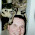## Thursday, September 29, 2011

### Given a set of integers select the minimum set with sum greater than k

Complexity? here is the code

#### 1 comment:

1.assuming numbers = 1, 3, 4, 11 and want to find minimum set with sum > k = 14 you can perhaps use knapsack pseudo-polynomial solution on the negated numbers, e.g.
neg.numbers = -1, -3, -4, -11 and find the knapsack solution with limit k=-15 - this is likely to give -15 = with the numbers -4, -11.
Dynamic programming pseudo solution has complexity O(NW), but looks like there has been recent work (2010) on improving it:
http://ieeexplore.ieee.org/xpl/freeabs_all.jsp?arnumber=5564469